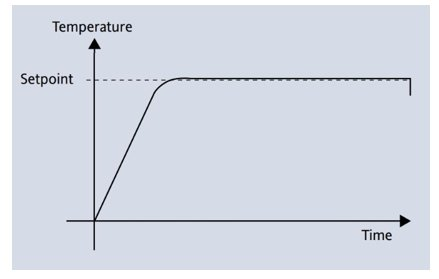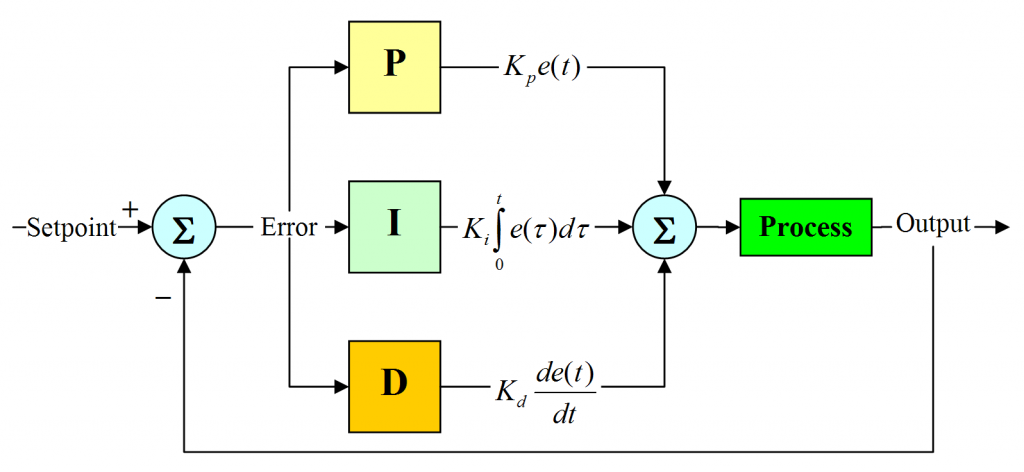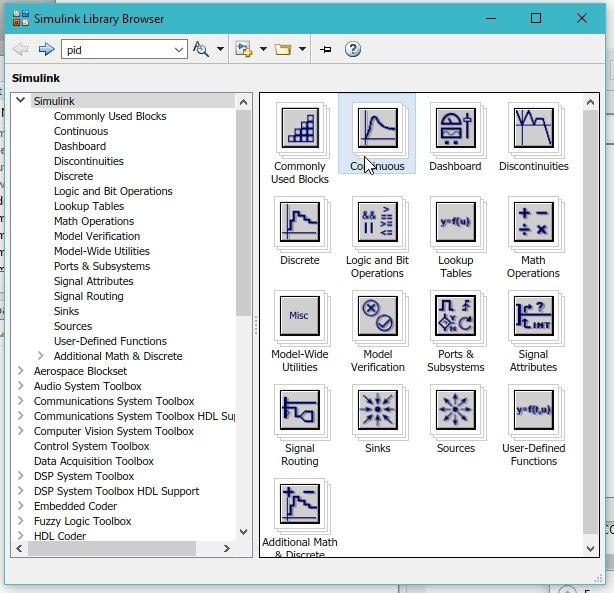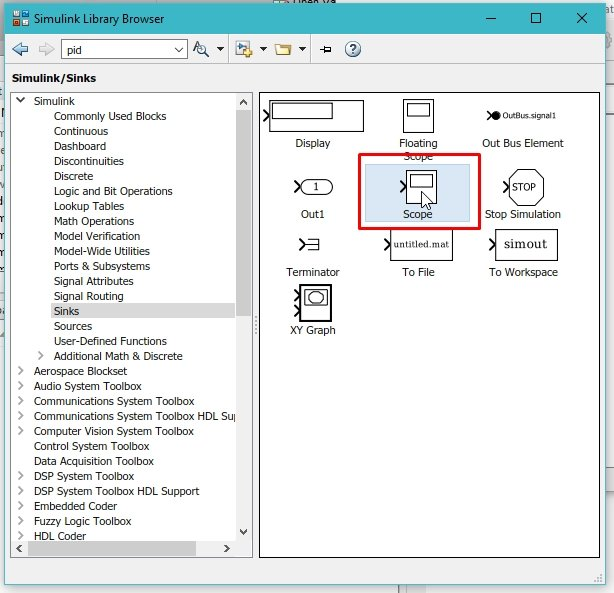# PID controller design using Simulink MATLAB : Tutorial 3

In this tutorial, a simple PID (Proportional Integral Derivative) is designed using MATLABs’ Simulink. At the start a brief and comprehensive introduction to a PID controller is given and a simple block diagram which can help you to implement a PID controller on a simple input on your own. After that a simple example is provided in which the controller is designed using Simulink. We can design a PID controller on Simulink in two different ways, each of the two ways is implemented and after the implementation the results from both the methods are compared. At the end a simple exercise is provided regarding the concepts and blocks used in this tutorial. You may also like to check these tutorials on Simulink:

### Introduction to PID controller

PID (Proportional Integral Derivative) controllers are the most widely used controllers in industrial settings because of their ease of use and the satisfaction of performance they are capable to provide the user for a large number of processes. Although the cost/benefit ratio provided by these controllers is way more than provided by any other controller. Many techniques have been proposed for their design, because of their widespread use, for the tuning of the parameters of PID i.ie Kp, Ki and Kd and for the implementation of additional functionalities that improve their performance.Figure 1: PID control

Control loops are used almost everywhere now a day. Anytime we adjust our current works according to the results obtained in previous results we form a control loop. For example, when we feel cold and turn our heater on we form a feedback loop, and when we press the accelerator of a car whenever we are getting late we again for a control loop.Whenever we change make any change in environment by sensing the previous results of that process we form a closed control loop in our mind. Changing the speed of the car is one of the best examples. The block diagram of a simple PID controller is provided in the figure below,Figure 2: PID block diagram

### PID controller design using Simulink MATLAB

Lets’ now move towards a simple example regarding the working of a simple PID controller using Simulink. In Simulink a PID controller can be designed using two different methods. Simulink contains a block named PID in its library browser. We can implement the PID controller by either using the built in PID block or we can design our own PID controller using the block diagram in figure 2. The results of both of them are however not the same as you will see shortly. Lets’ now begin with the programming part. Open MATLAB and then Simulink as we have down in previous tutorials. After that open the library browser and from the library browser select the continuous sub block as shown in the figure below,Figure 3: Continuous sub block

Figure 4: PID controller

Figure 5: Sources

Figure 6: Step response

Figure 7: Sink

• From the sinks subsection select the scope which will be used to display the output. You can also access the scope block from the commonly used blocks section in the library browser. Also if you do not know where exactly the block is placed in the library browser of simulink you can also search the block by typing its name in the search bar in the library browser. Refer to the figure below to see the scope block selected from the sinks section.Figure 8: Scope

Figure 9: Transfer function

Figure 10: Sum block

Figure 11: Placed components

Figure 12: List of signs

Figure 13: Transfer function

Figure 14: Step response

Figure 15: PID parameters

Figure 16: Block diagram

Figure 17: Model configuration

Figure 18: Model configuration steps

Figure 19: Running

Figure 20: Output

Figure 21: Gain blocks

Figure 22: Derivative block

Figure 23: PID controller

Figure 24: Complete block diagram

Figure 25: Output

The output is slightly underdamped and it can be adjusted by tuning the gains of the PID controller and it is left as an exercise for the reader.

Exercise:

• Tune the values of Kp, Ki, and Kd try to make the step response of the transfer function critically damped.

### 1 thought on “PID controller design using Simulink MATLAB : Tutorial 3”

1. hello, is there a way you can provide the code to run the model above please? I would appreciate